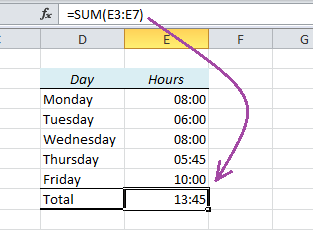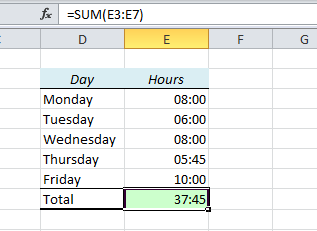# How to Summarize Hours in Excel and Show the Right ResultSometimes Excel doesn’t seem to add up the hours correctly. In this table I have made a table of an employee’s working hours and used the SUM function to add them together. Look what happens:

The sum is 13:45 instead of the expected 37:45. That’s 24 hours less than we wanted!

Here’s why: The result is actually 1 day, 13 hours and 45 minutes, but when you use the SUM function, Excel assumes that you want to keep the same format as above, which only shows hours and minutes.

How to fix it:1. Open the Format Cells window (Ctrl+1) and choose Custom.
2. If you want to show the result in days, hours and minutes, the format should be dd:hh:mm. Result: 01:13:45
3. If you want to show the result in hours and minutes, the format should be [h]:mm. Result: 37:45

As you see above, the square bracket tells Excel to summarize that particular time unit, so if you want to show the time in minutes, enter the format [m]. It works for seconds too: [s]

Other Useful Tricks:

Are you using a non-English version of Excel? Click here for translations of the 100 most common functions.